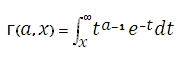# SQL Server incomplete gamma function

Updated: 9 August 2010

Use GAMMAINC to calculate the incomplete gamma function. This is the equivalent of the Gamma[a,z] function in Mathematica.Syntax
SELECT [wctStatistics].[wct].[GAMMAINC] (
<@A, float,>
,<@X, float,>)
Arguments
@A
a value to be passed to the function. @A is an expression of type float or of a type that can be implicitly converted to float
@X
a value to be passed to the function. @X is an expression of type float or of a type that can be implicitly converted to float
Return Types
float
Remarks
·         If @A ≤ 0 or @X ≤ 0, GAMMAINC returns an error.
Examples

select
wct.GAMMAINC(2, 2)

This produces the following result
----------------------
0.406005850189806

(1 row(s) affected)

### SupportCopyright 2008-2020 Westclintech LLC         Privacy Policy        Terms of Service